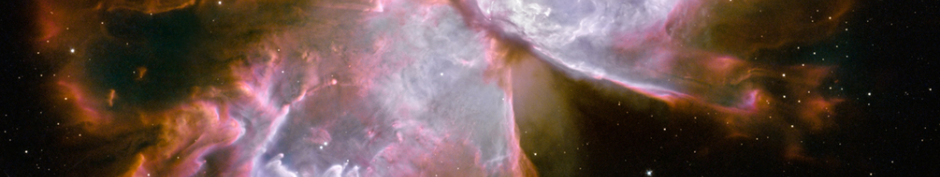The Physical Electron, The Neutron Inner Orbit Model and The Emergent Universe

The Physical Electron

Abstract

﻿

The electron is generally regarded as an elementary particle with no known components or substructure, due in part to the inability to measure its size. This measurement problem raises the possibility that this current characterization could be due to unusual operational and structural characteristics of the electron. This possibility provided the rationale for the current work which is the evaluation of a potential causative model of the electron based on the interactions of two postulated fundamental massless particles. It is shown that this model, when evaluated in the context of the fundamental constants, defines and evaluates properties of the massless particles and additional properties of the electron and proton. The equations and numerical values for the fundamental constants are presented in terms of the properties of the two massless particles and the new properties of the electron and proton. The fact that these equations accurately calculate the known values of the fundamental constants and satisfy the equations of the geometric model suggests that the electron could possess an unusual dynamic physical structure.

The Neutron Inner Orbit Model

﻿Abstract

﻿

﻿The principal postulate for this analysis is that the neutron is actually a proton with an electron in an inner orbit near to the fully contracted proton. It was shown in the first article ("The Physical Electron") that the postulated physical models for the electron and proton are outer shells of C2 particles pulsating 180º out of phase. The dynamic mechanisms associated with these electron and proton models for an inner electron orbit include special relativity, quantization by inverse integers, the modified Coulomb’s law, and shared C2 particles (shared mass equivalent energy (mee)) between the proton and electron. These effects are expressed in the appropriate governing equations which are the neutron energy equation, the electron force balance equation, the geometric relation between the descriptive radii, and the electron angular momentum equation. The simultaneous solution of these equations for the special case which utilizes the experimentally determined neutron excess mee as input,
results in a calculated orbit
that is the closest possible orbit to the proton without exceeding the speed of light for the electron orbital
velocity. The solution of the governing equations for the general case which utilizes the orbital index number as input, results in a calculated value of the neutron excess mee that matches the experimental value for the same orbital index number.

The Emergent Universe

Abstract

﻿

This is the third of three articles that present the concept that all mechanisms of the universe have emerged from the interactions of two fundamental massless particles under specific conditions. The first article ("The Physical Electron") identified properties of the massless particles and additional properties of the electron and proton that, when combined with a geometric model, result in equations that accurately compute the fundamental constants and electron and proton properties. The second article ("The Neutron Inner Orbit Model") developed a neutron inner orbit model that accurately calculates the neutron excess mass equivalent energy. The success of these models in reproducing the experimental data implies that the universe has emerged from the interactions of two massless particles that successively create new conditions and interactions resulting in increasing complex systems. This article investigates some the implied emergent mechanisms that underlie these systems. The mechanisms investigated are those responsible for dynamic electrons and protons, the characteristics of light, space as a flexible and responsive grid system, gravity, magnetism, particle-wave duality, relativistic mass, mass equivalent energy and momentum, time dilation, length contraction, and characteristics of the universe (cosmology). The conclusion is that the implied mechanisms that stem from the successful models developed in the first two articles result in a coherent and comprehensive depiction of the emergent universe.

## Where to Find Us:

Gene Callens
204 Lilinda Dr 204

Ruston, LA 71270

Phone: 318 2512928